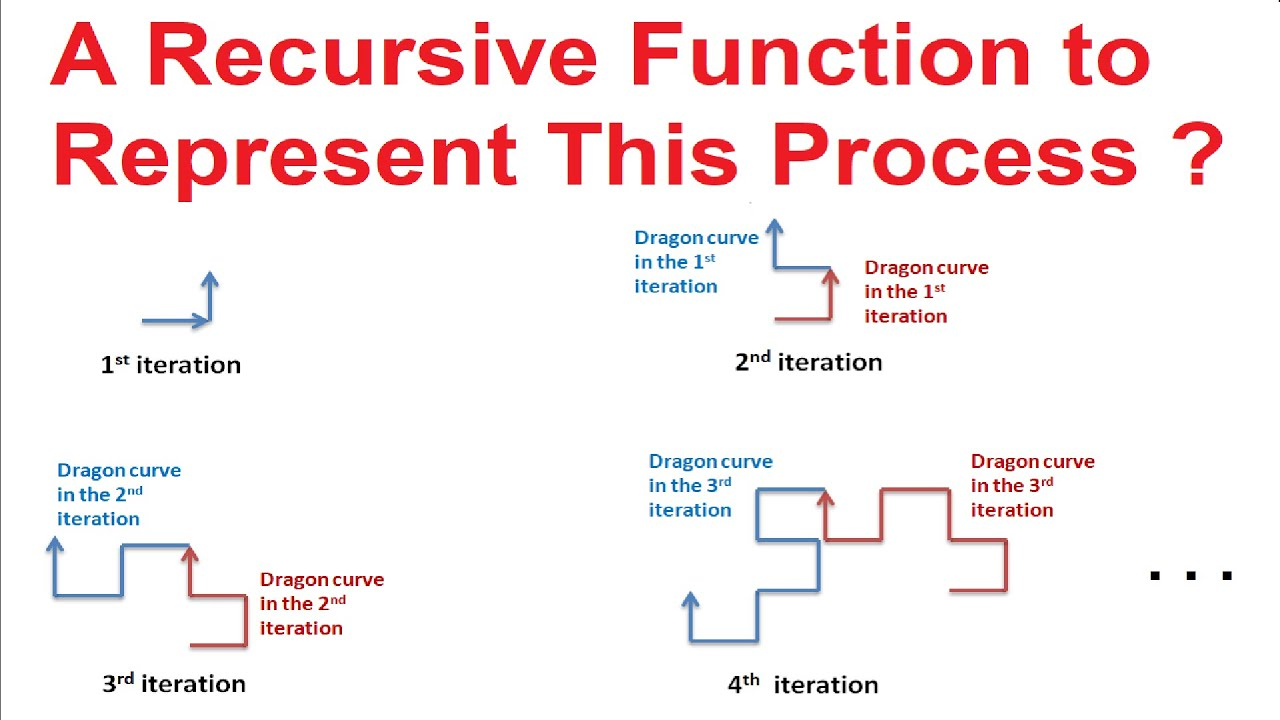# Coding With Music: Python Turtle Graphics-Recursive Construction of Dragon Curve #9Coding With Music: Python Turtle Graphics-Recursive Construction of Dragon Curve #9. This tutorial presents a recursive construction of a dragon curve.

ドラゴン曲線は、NASAの物理学者のJ. E. Heighwayによって初めて研究され、 「数学ゲーム（Mathematical Games」で紹介されたものである。 This tutorial presents a recursive construction of a dragon curve.

1) Presents algorithm of the recursive dragon curve. 2) Presents an efficient Python turtle graphics code for the algorithm.

## top 30 Python Tips and Tricks for Beginners

In this post, we'll learn top 30 Python Tips and Tricks for Beginners